Courses

# Centre of mass, Motion Of A Group Of Particles Physics Notes | EduRev

## Physics : Centre of mass, Motion Of A Group Of Particles Physics Notes | EduRev

The document Centre of mass, Motion Of A Group Of Particles Physics Notes | EduRev is a part of the Physics Course Basic Physics for IIT JAM.
All you need of Physics at this link: Physics

The word particle has been used in this article to signify an object whose entire mass is concentrated at a point in space. In the real world, however, there are no particles of this kind. All real bodies have sizes and shapes. Furthermore, as Newton believed and is now known, all bodies are in fact compounded of smaller bodies called atoms. Therefore, the science of mechanics must deal not only with particles but also with more complex bodies that may be thought of as collections of particles.
To take a specific example, the orbit of a planet around the Sun was discussed earlier as if the planet and the Sun were each concentrated at a point in space. In reality, of course, each is a substantial body. However, because each is nearly spherical in shape, it turns out to be permissible, for the purposes of this problem, to treat each body as if its mass were concentrated at its centre. This is an example of an idea that is often useful in discussing bodies of all kinds: the centre of mass. The centre of mass of a uniform sphere is located at the centre of the sphere. For many purposes (such as the one cited above) the sphere may be treated as if all its mass were concentrated at its centre of mass.
To extend the idea farther, consider Earth and the Sun not as two separate bodies but as a single system of two bodies interacting with one another by means of the force of gravity. In the previous discussion of circular orbits, the Sun was assumed to be at rest at the centre of the orbit, but, according to Newton’s third law, it must actually be accelerated by a force due to Earth that is equal and opposite to the force that the Sun exerts on Earth. In other words, considering only the Sun and Earth (ignoring, for example, all the other planets), if MS and ME are, respectively, the masses of the Sun and Earth, and if aS and aE are their respective accelerations, then combining Newton’s second and third laws results in the equation MSaS = −MEaE. Writing each a as dv/dt, this equation is easily manipulated to give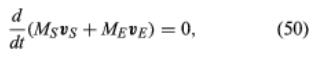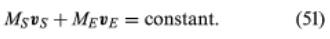This remarkable result means that, as Earth orbits the Sun and the Sun moves in response to Earth’s gravitational attraction, the entire two-body system has constant linear momentum, moving in a straight line at constant speed. Without any loss of generality, one can imagine observing the system from a frame of reference moving along with that same speed and direction. This is sometimes called the centre-of-mass frame. In this frame, the momentum of the two-body system—i.e., the constant in equation (51)—is equal to zero. Writing each of the v’s as the corresponding dr/dt, equation (51) may be expressed in the form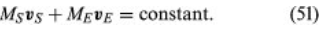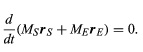(52)

Thus, MSrS and MErE are two vectors whose vector sum does not change with time. The sum is defined to be the constant vector MR, where M is the total mass of the system and equals MS + ME. Thus,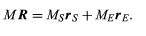(53)

This procedure defines a constant vector R, from any arbitrarily chosen point in space. The relation between vectors R, rS, and rE is shown in Figure 11. The fact that R is constant (although rS and rE are not constant) means that, rather than Earth orbiting the Sun, Earth and the Sun are both orbiting an imaginary point fixed in space. This point is known as the centre of mass of the two-body system.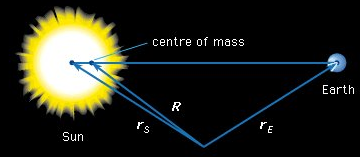Figure: The centre of mass of the two-body Earth-Sun system

Knowing the masses of the two bodies (MS = 1.99 × 1030 kilograms, ME = 5.98 × 1024 kilograms), it is easy to find the position of the centre of mass. The origin of the coordinate system may be chosen to be located at the centre of mass merely by defining R = 0. Then rS = (ME/MS) rE ≈ 450 kilometres, when rE is rounded to 1.5 × 108 km. A few hundred kilometres is so small compared to rE that, for all practical purposes, no appreciable error occurs when rS is ignored and the Sun is assumed to be stationary at the centre of the orbit.
With this example as a guide, it is now possible to define the centre of mass of any collection of bodies. Assume that there are N bodies altogether, each labeled with numbers ranging from 1 to N, and that the vector from an arbitrary origin to the ith body—where i is some number between 1 and N—is ri, as shown in Figure. Let the mass of the ith body be mi. Then the total mass of the N-body system is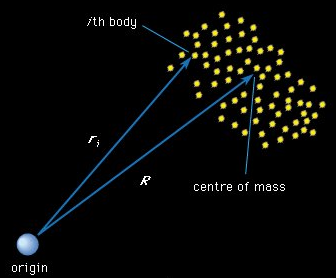Figure: The centre of mass of an N-body system.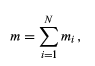(54)

and the centre of mass of the system is found at the end of a vector R given by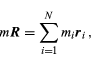(55)

as illustrated in Figure 12. This definition applies regardless of whether the N bodies making up the system are the stars in a galaxy, the atoms in a rigid body, larger and arbitrarily chosen segments of a rigid body, or any other system of masses. According to equation, the vector to the centre of mass of any system is a kind of weighted average of the vectors to all the components of the system.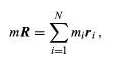(55)

As will be demonstrated in the sections that follow, the statics and dynamics of many complicated bodies or systems may often be understood by simply applying Newton’s laws as if the system’s mass were concentrated at the centre of mass.

Offer running on EduRev: Apply code STAYHOME200 to get INR 200 off on our premium plan EduRev Infinity!

,

,

,

,

,

,

,

,

,

,

,

,

,

,

,

,

,

,

,

,

,

,

,

,

;